# Maharashtra Board 12th Commerce Maths Solutions Chapter 8 Differential Equation and Applications Ex 8.1

Balbharati Maharashtra State Board Std 12 Commerce Statistics Part 1 Digest Pdf Chapter 8 Differential Equation and Applications Ex 8.1 Questions and Answers.

## Maharashtra State Board 12th Commerce Maths Solutions Chapter 8 Differential Equation and Applications Ex 8.1

Question 1.
Determine the order and degree of each of the following differential equations:
(i) $$\frac{d^{2} x}{d t^{2}}+\left(\frac{d x}{d t}\right)^{2}+8=0$$
Solution:
The given D.E. is $$\frac{d^{2} x}{d t^{2}}+\left(\frac{d x}{d t}\right)^{2}+8=0$$
This D.E. has highest order derivative $$\frac{d^{2} x}{d t^{2}}$$ with power 1.
∴ the given D.E. is of order 2 and degree 1.

(ii) $$\left(\frac{d^{2} y}{d x^{2}}\right)^{2}+\left(\frac{d y}{d x}\right)^{2}=a^{x}$$
Solution:
The given D.E. is $$\left(\frac{d^{2} y}{d x^{2}}\right)^{2}+\left(\frac{d y}{d x}\right)^{2}=a^{x}$$
This D.E. has highest order derivative $$\frac{d^{2} y}{d x^{2}}$$ with power 2.
∴ the given D.E. is of order 2 and degree 2.

(iii) $$\frac{d^{4} y}{d x^{4}}+\left[1+\left(\frac{d y}{d x}\right)^{2}\right]^{3}$$
Solution:
The given D.E. is $$\frac{d^{4} y}{d x^{4}}+\left[1+\left(\frac{d y}{d x}\right)^{2}\right]^{3}$$
This D.E. has highest order derivative $$\frac{d^{4} y}{d x^{4}}$$ with power 1.
∴ the given D.E. is of order 4 and degree 1.(iv) (y'”)2 + 2(y”)2 + 6y’ + 7y = 0
Solution:
The given D.E. is (y”‘)2 + 2(y”)2 + 6y’ + 7y = 0
This can be written as $$\left(\frac{d^{3} y}{d x^{3}}\right)^{2}+2\left(\frac{d^{2} y}{d x^{2}}\right)^{2}+6 \frac{d y}{d x}+7 y=0$$
This D.E. has highest order derivative $$\frac{d^{3} y}{d x^{3}}$$ with power 2.
∴ the given D.E. is of order 3 and degree 2.

(v) $$\sqrt{1+\frac{1}{\left(\frac{d y}{d x}\right)^{2}}}=\left(\frac{d y}{d x}\right)^{3 / 2}$$
Solution:
The given D.E. is $$\sqrt{1+\frac{1}{\left(\frac{d y}{d x}\right)^{2}}}=\left(\frac{d y}{d x}\right)^{3 / 2}$$
On squaring both sides, we get
$$1+\frac{1}{\left(\frac{d y}{d x}\right)^{2}}=\left(\frac{d y}{d x}\right)^{3}$$
∴ $$\left(\frac{d y}{d x}\right)^{2}+1=\left(\frac{d y}{d x}\right)^{5}$$
This D.E. has highest order derivative $$\frac{d y}{d x}$$ with power 5.
∴ the given D.E. is of order 1 and degree 5.

(vi) $$\frac{d y}{d x}=7 \frac{d^{2} y}{d x^{2}}$$
Solution:
The given D.E. is $$\frac{d y}{d x}=7 \frac{d^{2} y}{d x^{2}}$$
This D.E. has highest order derivative $$\frac{d^{2} y}{d x^{2}}$$ with power 1.
∴ the given D.E. is of order 2 and degree 1.

(vii) $$\left(\frac{d^{3} y}{d x^{3}}\right)^{1 / 6}=9$$
Solution:
The given D.E. is $$\left(\frac{d^{3} y}{d x^{3}}\right)^{1 / 6}=9$$
i.e., $$\frac{d^{3} y}{d x^{3}}=9^{6}$$
This D.E. has highest order derivative $$\frac{d^{3} y}{d x^{3}}$$ with power 1.
∴ the given D.E. is of order 3 and degree 1.Question 2.
In each of the following examples, verify that the given function is a solution of the corresponding differential equation: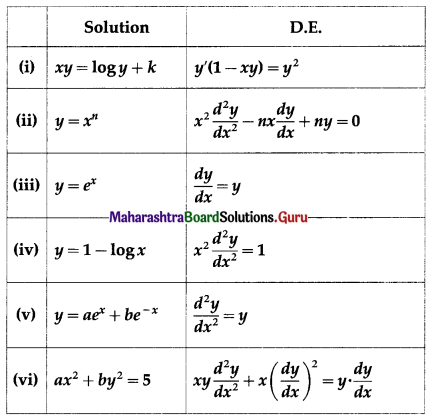Solution:
(i) xy = log y + k
Differentiating w.r.t. x, we get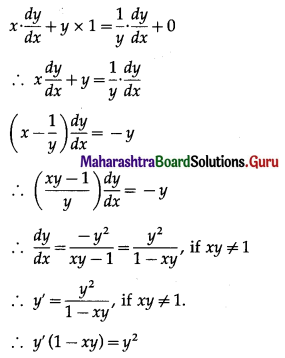Hence, xy = log y + k is a solution of the D.E. y'(1 – xy) = y2.

(ii) y = xn
Differentiating twice w.r.t. x, we get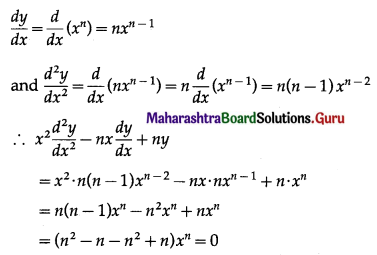This shows that y = xn is a solution of the D.E.
$$x^{2} \frac{d^{2} y}{d x^{2}}-n x \frac{d y}{d x}+n y=0$$

(iii) y = ex
Differentiating w.r.t. x, we get
$$\frac{d y}{d x}$$ = ex = y
Hence, y = ex is a solution of the D.E. $$\frac{d y}{d x}$$ = y.(iv) y = 1 – log x
Differentiating w.r.t. x, we get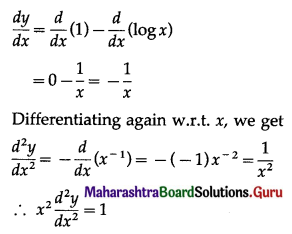Hence, y = 1 – log x is a solution of the D.E.
$$x^{2} \frac{d^{2} y}{d x^{2}}=1$$

(v) y = aex + be-x
Differentiating w.r.t. x, we get
$$\frac{d y}{d x}$$ = a(ex) + b(-e-x) = aex – be-x
Differentiating again w.r.t. x, we get
$$\frac{d^{2} y}{d x^{2}}$$ = a(ex) – b(-e-x)
= aex + be-x
= y
Hence, y = aex + be-x is a solution of the D.E. $$\frac{d^{2} y}{d x^{2}}$$ = y.(vi) ax2 + by2 = 5
Differentiating w.r.t. x, we get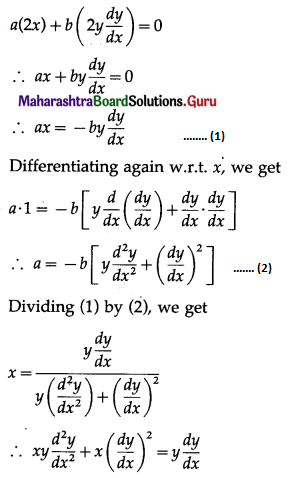Hence, ax2 + by2 = 5 is a solution of the D.E.
$$x y \frac{d^{2} y}{d x^{2}}+x\left(\frac{d y}{d x}\right)^{2}=y\left(\frac{d y}{d x}\right)$$